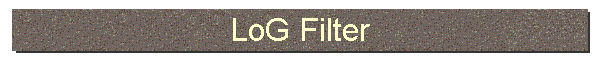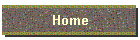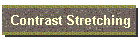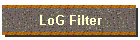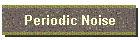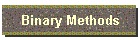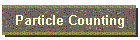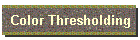Feb 14, 2001

Lab 2

Laplacian filters are derivative filters used to find areas of rapid change (edges) in images. Since derivative filters are very sensitive to noise, it is common to smooth the image (e.g., using a Gaussian filter) before applying the Laplacian. This two-step process is call the Laplacian of Gaussian (LoG) operation.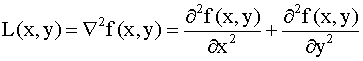There are different ways to find an approximate discrete convolution kernal that approximates the effect of the Laplacian. A possible kernel is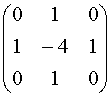This is called a negative Laplacian because the central peak is negative. It is just as appropriate to reverse the signs of the elements, using -1s and a +4, to get a positive Laplacian. It doesn't matter.

To include a smoothing Gaussian filter, combine the Laplacian and Gaussian functions to obtain a single equation: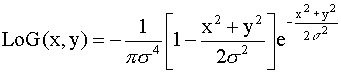A discrete kernel for the case of σ = 1.4 is given by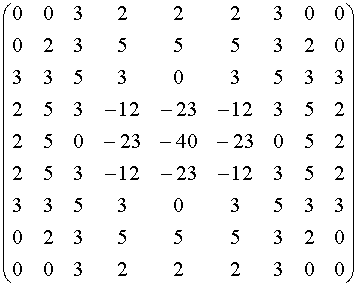The LoG operator takes the second derivative of the image. Where the image is basically uniform, the LoG will give zero. Wherever a change occurs, the LoG will give a positive response on the darker side and a negative response on the lighter side. At a sharp edge between two regions, the response will be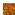zero away from the edgepositive just to one sidenegative just to the other sidezero at some point in between on the edge itself

When using the filter given above, or any other similar filter, the output can contain values that are quite large and may be negative, so it is important to use an image type that supports negatives and a large range, and then scale the output. (ImageJ has a 32-bit floating point representation). Alternatively, a scaling factor can be used on the filter to restrict the range of values.

Generate a LoG filter with Gaussian σ = 1.0, using a 7x7 kernel. Apply this filter to the image given below.

What can you say about the output image? Now combine (subtract) the two images in an effort to sharpen the original image. You may have to scale the filtered image before combining the two images. Also, you may have to translate the filtered image by half the width of the convolution kernel in both the x and y directions in order to register the images correctly. A little experimentation may be required. Save the result you think is your best effort

The enhancement sharpens the edges but also increases noise. If the original image is filtered with a simple Laplacian (a LoG filter with a very narrow Gaussian), the resulting output is rather noisy. Combining this output with the original will give a noisy result. On the other hand, using a larger σ for the Gaussian will reduce the noise, but the sharpening effect will be reduced. Life is full of compromises.